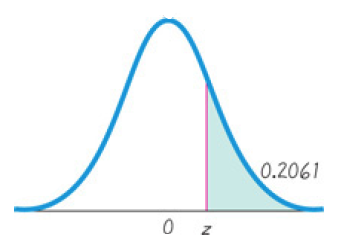×
Get Full Access to Elementary Statistics - 12 Edition - Chapter 6.2 - Problem 16bsc
Get Full Access to Elementary Statistics - 12 Edition - Chapter 6.2 - Problem 16bsc

×

# Standard Normal Distribution. In Exercises, | Ch 6.2 - 16BSCISBN: 9780321836960 18

## Solution for problem 16BSC Chapter 6.2

Elementary Statistics | 12th Edition

• Textbook Solutions
• 2901 Step-by-step solutions solved by professors and subject experts
• Get 24/7 help from StudySoup virtual teaching assistantsElementary Statistics | 12th Edition

4 5 1 247 Reviews
16
0
Problem 16BSC

Standard Normal Distribution. In Exercises 13–16, find the indicated z score. The graph depicts the standard normal distribution of bone density scores with mean 0 and standard deviation 1.Step-by-Step Solution:

Step 1 of 1The graph depicts the standard normal distribution of bone density scores with mean 0 and standard deviation 1.

From Area Under Normal Curve table we get,

1 - 0.2061 = 0.7939

The z-score corresponding to 0.7939 is 0.82.

Hence, the indicated z score is 0.82.

Step 2 of 1

##### ISBN: 9780321836960

Unlock Textbook Solution